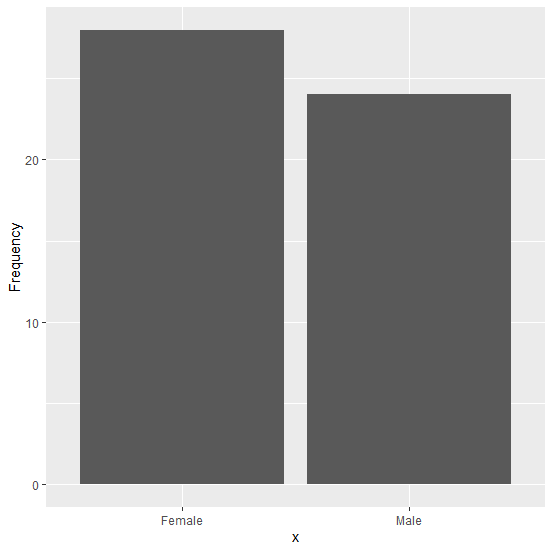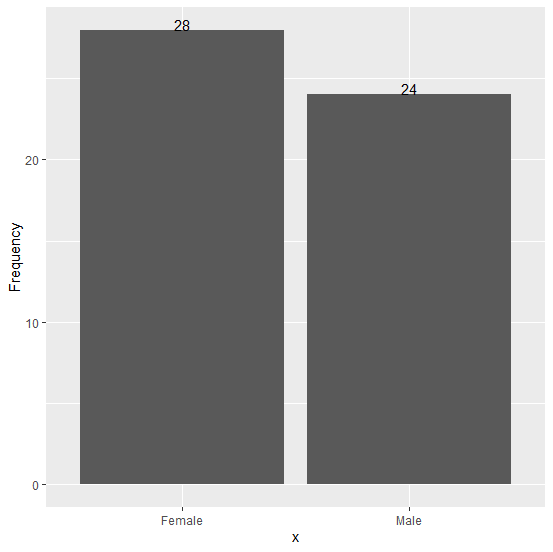# How to create a bar plot in R with label of bars on top of the bars using ggplot2?

There are multiple ways to represent a chart, specifically a bar plot is represented with so many variations. We can also include bar labels in a bar plot so that the viewer can easily understand the frequency of the categories for bars. To put the labels on top of the bars in a bar plot we can use vjust = 0 with geom_text in ggplot2.

## Example

Live Demo

Consider the below data frame −

df<-data.frame(x=factor(c("Male","Female")),Frequency=c(24,28))
df

## Output

  x Frequency
1 Male   24
2 Female 28

Loading ggplot2 and creating the simple bar plot without bar labels −

library(ggplot2)
ggplot(df,aes(x,Frequency))+geom_bar(stat="identity")

## OutputCreating the bar plot with bars label −

ggplot(df,aes(x,Frequency))+geom_bar(stat="identity")+
+ geom_text(aes(label=Frequency),vjust=0)

## Output